Plücker formulas

Formulas relating external characteristics, i.e. ones corresponding to projective imbeddings, and internal characteristics of algebraic varieties (cf. Algebraic variety). The oldest and best known numerical formulas in algebraic geometry are the Plücker formulas for a planar reduced and irreducible curve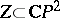, which has only ordinary double and cuspidal singular points. Letbe the degree of the curve, i.e. the number of points on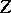on a straight line in general position in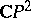, and let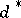be the class of, i.e. the number of straight lines tangent toat non-singular points and passing through a given fixed point in general position in. The two basic Plücker formulas are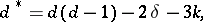(1)(2)

whereis the genus of the non-singular resolutionof(cf. Resolution of singularities),is the number of ordinary double points andis the number of cuspidal points. Formula (1) reduces to the form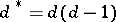ifis a non-singular curve.

Other classical Plücker formulas follow from (1) and (2) by duality. Ifis not a straight line, then the curve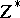dual tois defined as the closure of the set of tangents to, considered as points in the dual plane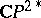. A theorem due to J. Plücker  states that the bi-dual curvecoincides with. If it is assumed thathas onlyordinary double points andcuspidal singular points, the formulas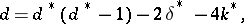(1ast)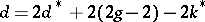(2ast)

are obtained. The number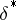can be interpreted also as the number of bitangents to, i.e. straight lines that touchat precisely two different non-singular points with contact of order two, while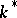is the number of points of inflection.

The four formulas (1), (2), (1ast), and (2ast) are not independent: the fourth follows from any other three. However, any three of them are independent. They also imply the following formulas: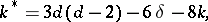(3)(3ast)

These formulas were derived by Plücker together with (1) and (1ast) in 1834–1839.

In the case of a ground field of finite characteristic, the Plücker formulas and the duality theorem are not always correct. For example, in characteristic 2 all the tangents to a conic pass through one point, which is called the strange point on the conic, so the dual curve is a straight line. In characteristic 3 there is a non-singular cubic with only three points of inflection, or even with one (according to the Plücker formulas there should be nine). With the correct interpretation of, (1) and (2) remain correct in all characteristics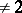; in characteristic 2 they must be replaced by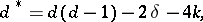(1astast)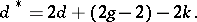(2astast)

There is a generalization of the Plücker formulas to curves inwith arbitrary singularities , and also to the case of hyperplanes in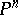.